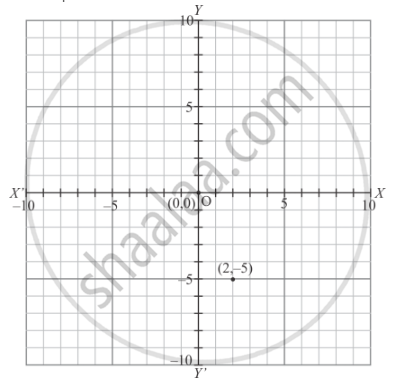# A Point Whose Abscissa and Ordinate Are 2 and −5 Respectively, Lies in - Mathematics

MCQ

A point whose abscissa and ordinate are 2 and −5 respectively, lies in

#### Solution

As shown in graph that a point whose abscissa and ordinate are  2 and  -5respectively lies in the fourth quadrant.Concept: Coordinate Geometry
Is there an error in this question or solution?

#### APPEARS IN

RD Sharma Mathematics for Class 9
Chapter 8 Co-ordinate Geometry
Exercise 8.2 | Q 4 | Page 7

Share